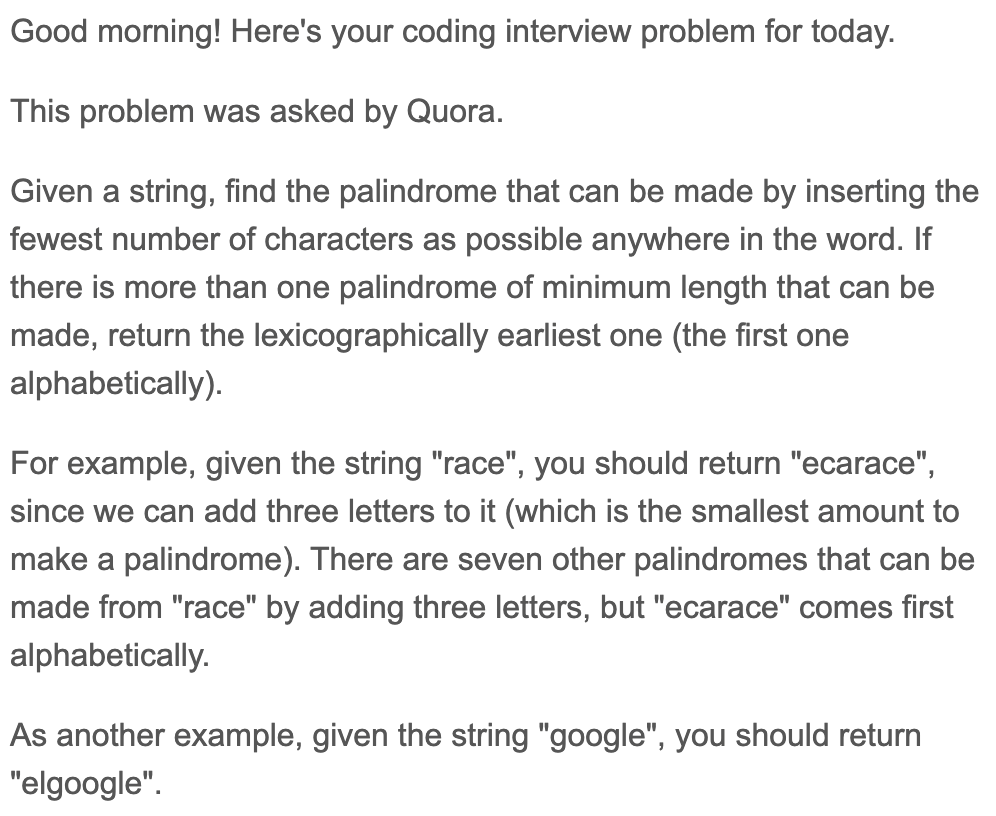# [daily coding] Minimum insertions to form a palindrome

victor·2021년 2월 24일
0

목록 보기
37/63

## problemGiven a string str, the task is to find the minimum number of characters to be inserted to convert it to palindrome.
Before we go further, let us understand with few examples:

ab: Number of insertions required is 1 i.e. bab
aa: Number of insertions required is 0 i.e. aa
abcd: Number of insertions required is 3 i.e. dcbabcd
abcda: Number of insertions required is 2 i.e. adcbcda which is same as number of insertions in the substring bcd(Why?).
abcde: Number of insertions required is 4 i.e. edcbabcde

## coding

const solution = (str) => {

return "";
}